# Max-flow min-cut theorem

The max-flow min-cut theorem is an important result in graph theory. It states that a weight of a minimum s-t cut in a graph equals the value of a maximum flow in a corresponding flow network.

As a consequence of this theorem, every max flow algorithm may be employed to solve the minimum s-t cut problem, and vice versa.

## Statement of the theorem

To every weighted s-t graph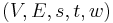$(V, E, s, t, w)$ we can associate a corresponding flow network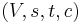$(V, s, t, c)$ in which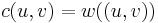$c(u, v) = w((u, v))$ if$(u, v) \in E$, and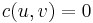$c(u, v) = 0$ otherwise. That is, the edges of the flow network are the same as the edges of the weighted s-t graph, with weights replaced by capacities.

Let$f$ be a maximum flow in a flow network$(V, s, t, c)$. Consider the set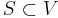$S \subset V$ consisting of vertices reachable from$s$ in the residual network corresponding to$f$. A minimum s-t cut of the corresponding weighted s-t graph$(V, E, s, t, w)$ is then given by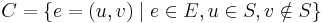$C = \{e = (u, v) \mid e \in E, u \in S, v \notin S \}$. Furthermore,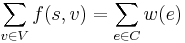$\sum_{v \in V} f(s, v) = \sum_{e \in C} w(e)$, that is, the max flow and the cut thus obtained are equal in value.

## Proof

We will first state and prove two Lemmas.

### Lemma 1

Let$f$ be a flow in a flow network$(V, s, t, c)$. Suppose we have a set$S \subset V$ such that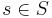$s \in S$ and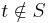$t \notin S$. Then the value of the flow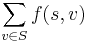$\sum_{v \in S} f(s, v)$ equals the total flow leaving$S$,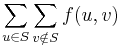$\sum_{u \in S} \sum_{v \notin S} f(u, v)$.

Proof:$\sum_{u \in S} \sum_{v \notin S} f(u, v)$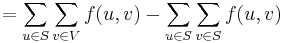$= \sum_{u \in S} \sum_{v \in V} f(u, v) - \sum_{u \in S} \sum_{v \in S} f(u, v)$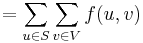$= \sum_{u \in S} \sum_{v \in V} f(u, v)$ (by skew symmetry)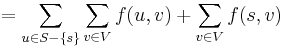$= \sum_{u \in S-\{s\}} \sum_{v \in V} f(u, v) + \sum_{v \in V} f(s, v)$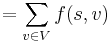$= \sum_{v \in V} f(s, v)$ (by flow conservation)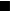$_\blacksquare$

Lemma 1 tells us that we can compute the value of a flow by arbitrarily partitioning the flow network into two sets$S$ and$T$, where$s \in S$ and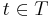$t \in T$, and adding only the flows along edges from$S$ to$T$. Intuitively, the conservation of flow implies that the total flow out of$S$ must equal the total flow emanating from the source; that flow has to get from$S$ to$T$ somehow.

### Lemma 2

Let$f$ be a flow in a flow network$(V, s, t, c)$. Let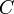$C$ be an s-t cut in the corresponding weighted s-t graph$(V, E, s, t, w)$. Then the value of$f$,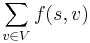$\sum_{v \in V} f(s, v)$, is less than or equal to the total weight of$C$,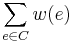$\sum_{e \in C} w(e)$.

Proof: Since$C$ is an s-t cut, in the weighted s-t graph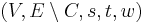$(V, E \setminus C, s, t, w)$, there is no path from$s$ to$t$. We can therefore consider the set$S$ of all vertices reachable from$s$ in this graph. Note that$t \notin S$. Applying Lemma 1, we find that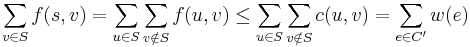$\sum_{v \in S} f(s, v) = \sum_{u \in S} \sum_{v \notin S} f(u, v) \leq \sum_{u \in S} \sum_{v \notin S} c(u, v) = \sum_{e \in C'} w(e)$.$_\blacksquare$

The idea behind Lemma 2 is simple. The value of a flow, which by Lemma 1 equals the total flow along edges out of$S$, cannot exceed the total capacity of such edges, but this is precisely the total weight of those edges in the corresponding weighted s-t graph. Lemma 2 is a useful result in its own right, telling us that every flow's value is less than or equal to every cut's weight.

### Main result

It suffices to show that$\sum_{v \in V} f(s, v) = \sum_{e \in C} w(e)$. The fact that the cut$C$ is minimal then follows immediately from Lemma 2.

Since the flow$f$ is maximal, there is no augmenting path in the residual network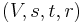$(V, s, t, r)$. Therefore,$t \notin S$. Now,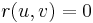$r(u, v) = 0$ for all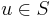$u \in S$ and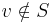$v \notin S$, by definition of$S$. Therefore,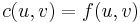$c(u, v) = f(u, v)$ for all such edges. By Lemma 1,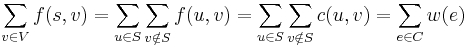$\sum_{v \in V} f(s, v) = \sum_{u \in S} \sum_{v \notin S} f(u, v) = \sum_{u \in S} \sum_{v \notin S} c(u, v) = \sum_{e \in C} w(e)$.$_\blacksquare$

## Discussion

In other words, we can solve the minimum s-t cut problem simply by converting all the edge weights to capacities and then finding a maximum flow in the resulting flow network. If we only want the weight of the min cut, we can simply find the value of the max flow, and be done with it. If we want to find the cut explicitly, then it is still straightforward to do so using the residual graph corresponding to the max flow. The "s part" of the s-t cut will consist of vertices reachable from$s$ in the residual graph, with the remainder the "t part". The edges we want to cut are the ones from the "s part" to the "t part".